• Difficulty Level : Easy
• Last Updated : 28 Mar, 2022

A linked list is a linear data structure, in which the elements are not stored at contiguous memory locations. The elements in a linked list are linked using pointers. In simple words, a linked list consists of nodes where each node contains a data field and a reference(link) to the next node in the list.

• Singly Linked List: It is the simplest type of linked list in which every node contains some data and a pointer to the next node of the same data type. The node contains a pointer to the next node means that the node stores the address of the next node in the sequence. A single linked list allows traversal of data only in one way. Below is the image for the same: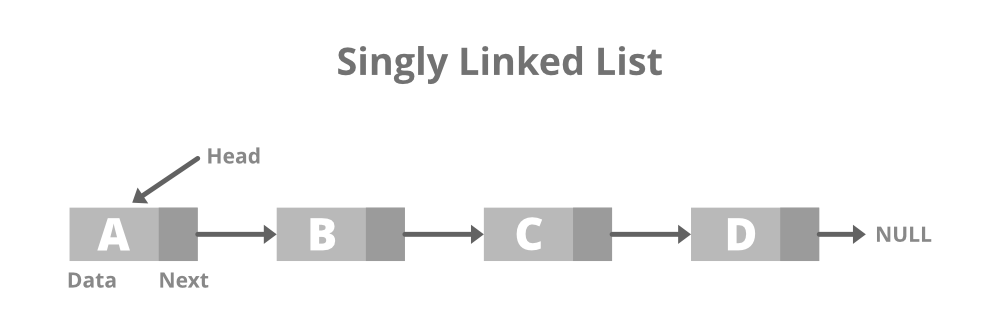• Structure of Singly Linked List:

## C++

 `// Node of a doubly linked list``class` `Node {``public``:``    ``int` `data;` `    ``// Pointer to next node in LL``    ``Node* next;``};`

## Java

 `// Node of a doubly linked list``static` `class` `Node``{``  ``int` `data;``  ` `   ``// Pointer to next node in LL``  ``Node next;``};` `//this code is contributed by shivani`

## Python3

 `# structure of Node``class` `Node:``    ``def` `__init__(``self``, data):``        ``self``.data ``=` `data``        ``self``.``next` `=` `None`

## C#

 `// Structure of Node``public` `class` `Node``{``    ``public` `int` `data;``    ` `    ``// Pointer to next node in LL``    ``public` `Node next;``};` `//this code is contributed by shivanisinghss2110`

## Javascript

 `// Node of a doubly linked list``class Node``{``    ``constructor()``    ``{``        ``this``.data=0;``        ` `        ``// Pointer to next node``        ``this``.next=``null``;``    ``}``}` `// This code is contributed by SHUBHAMSINGH10`
• Creation and Traversal of Singly Linked List:

## C++

 `// C++ program to illustrate creation``// and traversal of Singly Linked List` `#include ``using` `namespace` `std;` `// Structure of Node``class` `Node {``public``:``    ``int` `data;``    ``Node* next;``};` `// Function to print the content of``// linked list starting from the``// given node``void` `printList(Node* n)``{` `    ``// Iterate till n reaches NULL``    ``while` `(n != NULL) {` `        ``// Print the data``        ``cout << n->data << ``" "``;``        ``n = n->next;``    ``}``}` `// Driver Code``int` `main()``{``    ``Node* head = NULL;``    ``Node* second = NULL;``    ``Node* third = NULL;` `    ``// Allocate 3 nodes in the heap``    ``head = ``new` `Node();``    ``second = ``new` `Node();``    ``third = ``new` `Node();` `    ``// Assign data in first node``    ``head->data = 1;` `    ``// Link first node with second``    ``head->next = second;` `    ``// Assign data to second node``    ``second->data = 2;``    ``second->next = third;` `    ``// Assign data to third node``    ``third->data = 3;``    ``third->next = NULL;` `    ``printList(head);` `    ``return` `0;``}`

## Java

 `// Java program to illustrate``// creation and traversal of``// Singly Linked List``class` `GFG{` `// Structure of Node``static` `class` `Node``{``  ``int` `data;``  ``Node next;``};` `// Function to print the content of``// linked list starting from the``// given node``static` `void` `printList(Node n)``{``  ``// Iterate till n reaches null``  ``while` `(n != ``null``)``  ``{``    ``// Print the data``    ``System.out.print(n.data + ``" "``);``    ``n = n.next;``  ``}``}` `// Driver Code``public` `static` `void` `main(String[] args)``{``  ``Node head = ``null``;``  ``Node second = ``null``;``  ``Node third = ``null``;` `  ``// Allocate 3 nodes in``  ``// the heap``  ``head = ``new` `Node();``  ``second = ``new` `Node();``  ``third = ``new` `Node();` `  ``// Assign data in first``  ``// node``  ``head.data = ``1``;` `  ``// Link first node with``  ``// second``  ``head.next = second;` `  ``// Assign data to second``  ``// node``  ``second.data = ``2``;``  ``second.next = third;` `  ``// Assign data to third``  ``// node``  ``third.data = ``3``;``  ``third.next = ``null``;` `  ``printList(head);``}``}` `// This code is contributed by Princi Singh`

## C#

 `// C# program to illustrate``// creation and traversal of``// Singly Linked List``using` `System;` `class` `GFG{` `// Structure of Node``public` `class` `Node``{``    ``public` `int` `data;``    ``public` `Node next;``};` `// Function to print the content of``// linked list starting from the``// given node``static` `void` `printList(Node n)``{``    ` `    ``// Iterate till n reaches null``    ``while` `(n != ``null``)``    ``{``        ` `        ``// Print the data``        ``Console.Write(n.data + ``" "``);``        ``n = n.next;``    ``}``}` `// Driver Code``public` `static` `void` `Main(String[] args)``{``    ``Node head = ``null``;``    ``Node second = ``null``;``    ``Node third = ``null``;``    ` `    ``// Allocate 3 nodes in``    ``// the heap``    ``head = ``new` `Node();``    ``second = ``new` `Node();``    ``third = ``new` `Node();``    ` `    ``// Assign data in first``    ``// node``    ``head.data = 1;``    ` `    ``// Link first node with``    ``// second``    ``head.next = second;``    ` `    ``// Assign data to second``    ``// node``    ``second.data = 2;``    ``second.next = third;``    ` `    ``// Assign data to third``    ``// node``    ``third.data = 3;``    ``third.next = ``null``;``    ` `    ``printList(head);``}``}` `// This code is contributed by Amit Katiyar`

## Python3

 `# structure of Node``class` `Node:``    ``def` `__init__(``self``, data):``        ``self``.data ``=` `data``        ``self``.``next` `=` `None`  `class` `LinkedList:``    ``def` `__init__(``self``):``        ``self``.head ``=` `None``        ``self``.last_node ``=` `None``        ` `    ``# function to add elements to linked list``    ``def` `append(``self``, data):``        ``# if linked list is empty then last_node will be none so in if condition head will be created``        ``if` `self``.last_node ``is` `None``:``            ``self``.head ``=` `Node(data)``            ``self``.last_node ``=` `self``.head``        ``# adding node to the tail of linked list``        ``else``:``            ``self``.last_node.``next` `=` `Node(data)``            ``self``.last_node ``=` `self``.last_node.``next``            ` `# function to print the content of linked list``    ``def` `display(``self``):``        ``current ``=` `self``.head``      ``# traversing the linked list``        ``while` `current ``is` `not` `None``:``          ``# at each node printing its data``            ``print``(current.data, end``=``' '``)``           ``# giving current next node``            ``current ``=` `current.``next``        ``print``()`  `if` `__name__ ``=``=` `'__main__'``:``    ``L ``=` `LinkedList()``    ``# adding elements to the linked list``    ``L.append(``1``)``    ``L.append(``2``)``    ``L.append(``3``)``    ``L.append(``4``)``    ``# displaying elements of linked list``    ``L.display()`

## Javascript

 ``
Output
`1 2 3 `
• Doubly Linked List: A doubly linked list or a two-way linked list is a more complex type of linked list which contains a pointer to the next as well as the previous node in sequence, Therefore, it contains three parts are data, a pointer to the next node, and a pointer to the previous node. This would enable us to traverse the list in the backward direction as well. Below is the image for the same: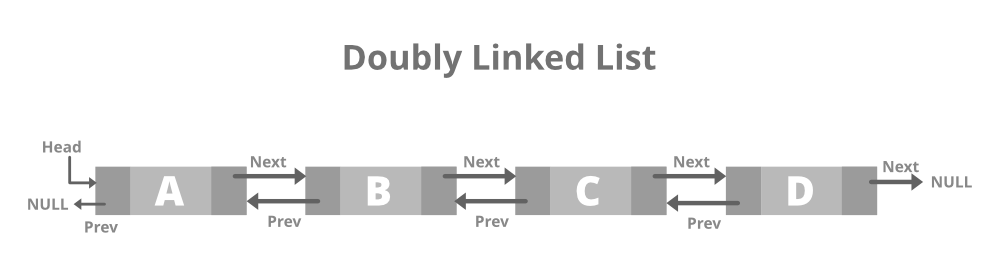• Structure of Doubly Linked List:

## C++

 `// Node of a doubly linked list``struct` `Node {``    ``int` `data;` `    ``// Pointer to next node in DLL``    ``struct` `Node* next;` `    ``// Pointer to the previous node in DLL``    ``struct` `Node* prev;``};`

## Java

 `// Doubly linked list``// node``static` `class` `Node``{``    ``int` `data;``    ` `    ``// Pointer to next node in DLL``    ``Node next;``    ` `    ``// Pointer to the previous node in DLL``    ``Node prev;``};` `// This code is contributed by shivani`

## Python3

 `# structure of Node``class` `Node:``    ``def` `__init__(``self``, data):``        ``self``.previous ``=` `None``        ``self``.data ``=` `data``        ``self``.``next` `=` `None`

## C#

 `// Doubly linked list``// node``public` `class` `Node``{``    ``public` `int` `data;``    ` `    ``// Pointer to next node in DLL``    ``public` `Node next;``    ` `    ``// Pointer to the previous node in DLL``    ``public` `Node prev;``};` `// This code is contributed by shivanisinghss2110`
• Creation and Traversal of Doubly Linked List:

## C++

 `// C++ program to illustrate creation``// and traversal of Doubly Linked List``#include ``using` `namespace` `std;` `// Doubly linked list node``class` `Node {``public``:``    ``int` `data;``    ``Node* next;``    ``Node* prev;``};` `// Function to push a new element in``// the Doubly Linked List``void` `push(Node** head_ref, ``int` `new_data)``{``    ``// Allocate node``    ``Node* new_node = ``new` `Node();` `    ``// Put in the data``    ``new_node->data = new_data;` `    ``// Make next of new node as``    ``// head and previous as NULL``    ``new_node->next = (*head_ref);``    ``new_node->prev = NULL;` `    ``// Change prev of head node to``    ``// the new node``    ``if` `((*head_ref) != NULL)``        ``(*head_ref)->prev = new_node;` `    ``// Move the head to point to``    ``// the new node``    ``(*head_ref) = new_node;``}` `// Function to traverse the Doubly LL``// in the forward & backward direction``void` `printList(Node* node)``{``    ``Node* last;` `    ``cout << ``"\nTraversal in forward"``         ``<< ``" direction \n"``;``    ``while` `(node != NULL) {` `        ``// Print the data``        ``cout << ``" "` `<< node->data << ``" "``;``        ``last = node;``        ``node = node->next;``    ``}` `    ``cout << ``"\nTraversal in reverse"``         ``<< ``" direction \n"``;``    ``while` `(last != NULL) {` `        ``// Print the data``        ``cout << ``" "` `<< last->data << ``" "``;``        ``last = last->prev;``    ``}``}` `// Driver Code``int` `main()``{``    ``// Start with the empty list``    ``Node* head = NULL;` `    ``// Insert 6.``    ``// So linked list becomes 6->NULL``    ``push(&head, 6);` `    ``// Insert 7 at the beginning. So``    ``// linked list becomes 7->6->NULL``    ``push(&head, 7);` `    ``// Insert 1 at the beginning. So``    ``// linked list becomes 1->7->6->NULL``    ``push(&head, 1);` `    ``cout << ``"Created DLL is: "``;``    ``printList(head);` `    ``return` `0;``}`

## Java

 `// Java program to illustrate``// creation and traversal of``// Doubly Linked List``import` `java.util.*;``class` `GFG{` `// Doubly linked list``// node``static` `class` `Node``{``  ``int` `data;``  ``Node next;``  ``Node prev;``};``  ` `static` `Node head_ref;``  ` `// Function to push a new``// element in the Doubly``// Linked List``static` `void` `push(``int` `new_data)``{``  ``// Allocate node``  ``Node new_node = ``new` `Node();` `  ``// Put in the data``  ``new_node.data = new_data;` `  ``// Make next of new node as``  ``// head and previous as null``  ``new_node.next = head_ref;``  ``new_node.prev = ``null``;` `  ``// Change prev of head node to``  ``// the new node``  ``if` `(head_ref != ``null``)``    ``head_ref.prev = new_node;` `  ``// Move the head to point to``  ``// the new node``  ``head_ref = new_node;``}` `// Function to traverse the``// Doubly LL in the forward``// & backward direction``static` `void` `printList(Node node)``{``  ``Node last = ``null``;` `  ``System.out.print(``"\nTraversal in forward"` `+``                   ``" direction \n"``);``  ``while` `(node != ``null``)``  ``{``    ``// Print the data``    ``System.out.print(``" "` `+  node.data +``                     ``" "``);``    ``last = node;``    ``node = node.next;``  ``}` `  ``System.out.print(``"\nTraversal in reverse"` `+``                   ``" direction \n"``);``  ` `  ``while` `(last != ``null``)``  ``{``    ``// Print the data``    ``System.out.print(``" "` `+  last.data +``                     ``" "``);``    ``last = last.prev;``  ``}``}` `// Driver Code``public` `static` `void` `main(String[] args)``{``  ``// Start with the empty list``  ``head_ref = ``null``;` `  ``// Insert 6.``  ``// So linked list becomes``  ``// 6.null``  ``push(``6``);` `  ``// Insert 7 at the beginning.``  ``// So linked list becomes``  ``// 7.6.null``  ``push(``7``);` `  ``// Insert 1 at the beginning.``  ``// So linked list becomes``  ``// 1.7.6.null``  ``push(``1``);` `  ``System.out.print(``"Created DLL is: "``);``  ``printList(head_ref);``}``}` `// This code is contributed by Princi Singh`

## Python3

 `# structure of Node``class` `Node:``    ``def` `__init__(``self``, data):``        ``self``.previous ``=` `None``        ``self``.data ``=` `data``        ``self``.``next` `=` `None`  `class` `DoublyLinkedList:``    ``def` `__init__(``self``):``        ``self``.head ``=` `None``        ``self``.start_node ``=` `None``        ``self``.last_node ``=` `None` `    ``# function to add elements to doubly linked list``    ``def` `append(``self``, data):``        ``# is doubly linked list is empty then last_node will be none so in if condition head will be created``        ``if` `self``.last_node ``is` `None``:``            ``self``.head ``=` `Node(data)``            ``self``.last_node ``=` `self``.head``        ``# adding node to the tail of doubly linked list``        ``else``:``            ``new_node ``=` `Node(data)``            ``self``.last_node.``next` `=` `new_node``            ``new_node.previous ``=` `self``.last_node``            ``new_node.``next` `=` `None``            ``self``.last_node ``=` `new_node` `    ``# function to printing and traversing the content of doubly linked list from left to right and right to left``    ``def` `display(``self``, ``Type``):``        ``if` `Type` `=``=` `'Left_To_Right'``:``            ``current ``=` `self``.head``            ``while` `current ``is` `not` `None``:``                ``print``(current.data, end``=``' '``)``                ``current ``=` `current.``next``            ``print``()``        ``else``:``            ``current ``=` `self``.last_node``            ``while` `current ``is` `not` `None``:``                ``print``(current.data, end``=``' '``)``                ``current ``=` `current.previous``            ``print``()`  `if` `__name__ ``=``=` `'__main__'``:``    ``L ``=` `DoublyLinkedList()``    ``L.append(``1``)``    ``L.append(``2``)``    ``L.append(``3``)``    ``L.append(``4``)``    ``L.display(``'Left_To_Right'``)``    ``L.display(``'Right_To_Left'``)`

## C#

 `// C# program to illustrate``// creation and traversal of``// Doubly Linked List``using` `System;` `class` `GFG{` `// Doubly linked list``// node``public` `class` `Node``{``  ``public` `int` `data;``  ``public` `Node next;``  ``public` `Node prev;``};``  ` `static` `Node head_ref;``  ` `// Function to push a new``// element in the Doubly``// Linked List``static` `void` `push(``int` `new_data)``{``  ` `  ``// Allocate node``  ``Node new_node = ``new` `Node();` `  ``// Put in the data``  ``new_node.data = new_data;` `  ``// Make next of new node as``  ``// head and previous as null``  ``new_node.next = head_ref;``  ``new_node.prev = ``null``;` `  ``// Change prev of head node to``  ``// the new node``  ``if` `(head_ref != ``null``)``    ``head_ref.prev = new_node;` `  ``// Move the head to point to``  ``// the new node``  ``head_ref = new_node;``}` `// Function to traverse the``// Doubly LL in the forward``// & backward direction``static` `void` `printList(Node node)``{``  ``Node last = ``null``;` `  ``Console.Write(``"\nTraversal in forward"` `+``                ``" direction \n"``);``  ` `  ``while` `(node != ``null``)``  ``{``    ` `    ``// Print the data``    ``Console.Write(``" "` `+  node.data +``                  ``" "``);``    ``last = node;``    ``node = node.next;``  ``}` `  ``Console.Write(``"\nTraversal in reverse"` `+``                ``" direction \n"``);``  ` `  ``while` `(last != ``null``)``  ``{``    ` `    ``// Print the data``    ``Console.Write(``" "` `+  last.data +``                  ``" "``);``    ``last = last.prev;``  ``}``}` `// Driver Code``public` `static` `void` `Main(String[] args)``{``  ` `  ``// Start with the empty list``  ``head_ref = ``null``;` `  ``// Insert 6.``  ``// So linked list becomes``  ``// 6.null``  ``push(6);` `  ``// Insert 7 at the beginning.``  ``// So linked list becomes``  ``// 7.6.null``  ``push(7);` `  ``// Insert 1 at the beginning.``  ``// So linked list becomes``  ``// 1.7.6.null``  ``push(1);` `  ``Console.Write(``"Created DLL is: "``);``  ``printList(head_ref);``}``}` `// This code is contributed by Amit Katiyar`
Output
```Created DLL is:
Traversal in forward direction
1  7  6
Traversal in reverse direction
6  7  1```
• Circular Linked List: A circular linked list is that in which the last node contains the pointer to the first node of the list. While traversing a circular linked list, we can begin at any node and traverse the list in any direction forward and backward until we reach the same node we started. Thus, a circular linked list has no beginning and no end. Below is the image for the same: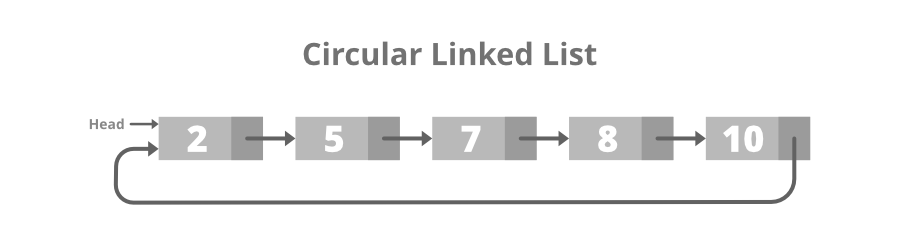• Structure of Circular Linked List:

## C++

 `// Structure for a node``class` `Node {``public``:``    ``int` `data;` `    ``// Pointer to next node in CLL``    ``Node* next;``};`

## Java

 `// Structure for a node``static` `class` `Node``{``  ``int` `data;``  ` `  ``// Pointer to next node in CLL``  ``Node next;``};` `// This code is contributed by shivanisinghss2110`

## Python3

 `# structure of Node``class` `Node:``    ``def` `__init__(``self``, data):``        ``self``.data ``=` `data``        ``self``.``next` `=` `None`

## C#

 `// Structure for a node``public` `class` `Node``{``  ``public` `int` `data;``  ` `  ``// Pointer to next node in CLL``  ``public` `Node next;``};` `// This code is contributed by shivanisinghss2110`
• Creation and Traversal of Circular Linked List:

## C++

 `// C++ program to illustrate creation``// and traversal of Circular LL``#include ``using` `namespace` `std;` `// Structure for a node``class` `Node {``public``:``    ``int` `data;``    ``Node* next;``};` `// Function to insert a node at the``// beginning of Circular LL``void` `push(Node** head_ref, ``int` `data)``{``    ``Node* ptr1 = ``new` `Node();``    ``Node* temp = *head_ref;``    ``ptr1->data = data;``    ``ptr1->next = *head_ref;` `    ``// If linked list is not NULL then``    ``// set the next of last node``    ``if` `(*head_ref != NULL) {``        ``while` `(temp->next != *head_ref) {``            ``temp = temp->next;``        ``}``        ``temp->next = ptr1;``    ``}` `    ``// For the first node``    ``else``        ``ptr1->next = ptr1;` `    ``*head_ref = ptr1;``}` `// Function to print nodes in the``// Circular Linked List``void` `printList(Node* head)``{``    ``Node* temp = head;``    ``if` `(head != NULL) {``        ``do` `{` `            ``// Print the data``            ``cout << temp->data << ``" "``;``            ``temp = temp->next;``        ``} ``while` `(temp != head);``    ``}``}` `// Driver Code``int` `main()``{``    ``// Initialize list as empty``    ``Node* head = NULL;` `    ``// Created linked list will``    ``// be 11->2->56->12``    ``push(&head, 12);``    ``push(&head, 56);``    ``push(&head, 2);``    ``push(&head, 11);` `    ``cout << ``"Contents of Circular"``         ``<< ``" Linked List\n "``;``    ``printList(head);` `    ``return` `0;``}`

## Java

 `// Java program to illustrate``// creation and traversal of``// Circular LL``import` `java.util.*;``class` `GFG{` `// Structure for a``// node``static` `class` `Node``{``  ``int` `data;``  ``Node next;``};` `// Function to insert a node``// at the beginning of Circular``// LL``static` `Node push(Node head_ref,``                 ``int` `data)``{``  ``Node ptr1 = ``new` `Node();``  ``Node temp = head_ref;``  ``ptr1.data = data;``  ``ptr1.next = head_ref;` `  ``// If linked list is not``  ``// null then set the next``  ``// of last node``  ``if` `(head_ref != ``null``)``  ``{``    ``while` `(temp.next != head_ref)``    ``{``      ``temp = temp.next;``    ``}``    ``temp.next = ptr1;``  ``}` `  ``// For the first node``  ``else``    ``ptr1.next = ptr1;` `  ``head_ref = ptr1;``  ``return` `head_ref;``}` `// Function to print nodes in``// the Circular Linked List``static` `void` `printList(Node head)``{``  ``Node temp = head;``  ``if` `(head != ``null``)``  ``{``    ``do``    ``{``      ``// Print the data``      ``System.out.print(temp.data + ``" "``);``      ``temp = temp.next;``    ``} ``while` `(temp != head);``  ``}``}` `// Driver Code``public` `static` `void` `main(String[] args)``{``  ``// Initialize list as empty``  ``Node head = ``null``;` `  ``// Created linked list will``  ``// be 11.2.56.12``  ``head = push(head, ``12``);``  ``head = push(head, ``56``);``  ``head = push(head, ``2``);``  ``head = push(head, ``11``);` `  ``System.out.print(``"Contents of Circular"` `+``                   ``" Linked List\n "``);``  ``printList(head);``}``}` `// This code is contributed by gauravrajput1`

## Python3

 `# structure of Node``class` `Node:``    ``def` `__init__(``self``, data):``        ``self``.data ``=` `data``        ``self``.``next` `=` `None`  `class` `CircularLinkedList:``    ``def` `__init__(``self``):``        ``self``.head ``=` `None``        ``self``.last_node ``=` `None` `    ``# function to add elements to circular linked list``    ``def` `append(``self``, data):``        ``# is circular linked list is empty then last_node will be none so in if condition head will be created``        ``if` `self``.last_node ``is` `None``:``            ``self``.head ``=` `Node(data)``            ``self``.last_node ``=` `self``.head``        ``# adding node to the tail of circular linked list``        ``else``:``            ``self``.last_node.``next` `=` `Node(data)``            ``self``.last_node ``=` `self``.last_node.``next``            ``self``.last_node.``next` `=` `self``.head` `    ``# function to print the content of circular linked list``    ``def` `display(``self``):``        ``current ``=` `self``.head``        ``while` `current ``is` `not` `None``:``            ``print``(current.data, end``=``' '``)``            ``current ``=` `current.``next``            ``if` `current ``=``=` `self``.head:``                ``break``        ``print``()`  `if` `__name__ ``=``=` `'__main__'``:``    ``L ``=` `CircularLinkedList()``    ``L.append(``1``)``    ``L.append(``2``)``    ``L.append(``3``)``    ``L.append(``4``)``    ``L.display()`

## C#

 `// C# program to illustrate``// creation and traversal of``// Circular LL``using` `System;` `class` `GFG{` `// Structure for a``// node``public` `class` `Node``{``  ``public` `int` `data;``  ``public` `Node next;``};` `// Function to insert a node``// at the beginning of Circular``// LL``static` `Node push(Node head_ref,``                 ``int` `data)``{``  ``Node ptr1 = ``new` `Node();``  ``Node temp = head_ref;``  ``ptr1.data = data;``  ``ptr1.next = head_ref;``  ` `  ``// If linked list is not``  ``// null then set the next``  ``// of last node``  ``if` `(head_ref != ``null``)``  ``{``    ``while` `(temp.next != head_ref)``    ``{``      ``temp = temp.next;``    ``}``    ``temp.next = ptr1;``  ``}` `  ``// For the first node``  ``else``    ``ptr1.next = ptr1;` `  ``head_ref = ptr1;``  ``return` `head_ref;``}` `// Function to print nodes in``// the Circular Linked List``static` `void` `printList(Node head)``{``  ``Node temp = head;``  ` `  ``if` `(head != ``null``)``  ``{``    ``do``    ``{``      ` `      ``// Print the data``      ``Console.Write(temp.data + ``" "``);``      ``temp = temp.next;``    ``} ``while` `(temp != head);``  ``}``}` `// Driver Code``public` `static` `void` `Main(String[] args)``{``  ` `  ``// Initialize list as empty``  ``Node head = ``null``;``  ` `  ``// Created linked list will``  ``// be 11.2.56.12``  ``head = push(head, 12);``  ``head = push(head, 56);``  ``head = push(head, 2);``  ``head = push(head, 11);` `  ``Console.Write(``"Contents of Circular "` `+``                ``"Linked List\n "``);``  ` `  ``printList(head);``}``}` `// This code is contributed by gauravrajput1`
Output
```Contents of Circular Linked List
11 2 56 12```
• Doubly Circular linked list: A Doubly Circular linked list or a circular two-way linked list is a more complex type of linked-list that contains a pointer to the next as well as the previous node in the sequence. The difference between the doubly linked and circular doubly list is the same as that between a singly linked list and a circular linked list. The circular doubly linked list does not contain null in the previous field of the first node. Below is the image for the same: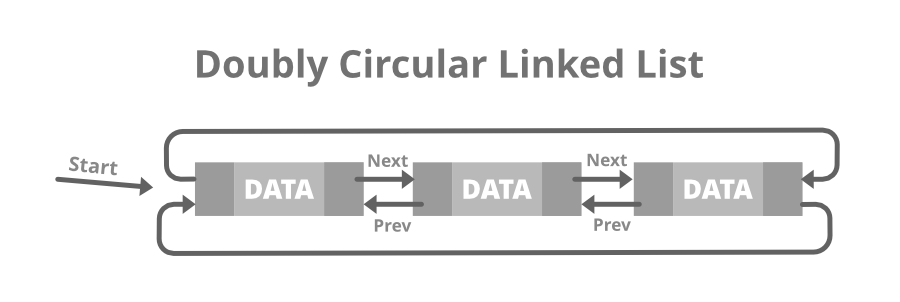• Structure of Doubly Circular Linked List:

## C++

 `// Node of doubly circular linked list``struct` `Node {` `    ``int` `data;` `    ``// Pointer to next node in DCLL``    ``struct` `Node* next;` `    ``// Pointer to the previous node in DCLL``    ``struct` `Node* prev;``};`

## Java

 `// Structure of a Node``static` `class` `Node``{``    ``int` `data;``    ` `    ``// Pointer to next node in DCLL``    ``Node next;``    ` `    ``// Pointer to the previous node in DCLL``    ``Node prev;``};` `//this code is contributed by shivanisinghss2110`

## Python3

 `# structure of Node``class` `Node:``    ``def` `__init__(``self``, data):``        ``self``.previous ``=` `None``        ``self``.data ``=` `data``        ``self``.``next` `=` `None`

## C#

 `// Structure of a Node``public` `class` `Node``{``    ``public`  `int` `data;``    ` `    ``// Pointer to next node in DCLL``    ``public` `Node next;``    ` `    ``// Pointer to the previous node in DCLL``    ``public` `Node prev;``};` `// This code is contributed by shivanisinghss2110`
• Creation and Traversal of Doubly Circular Linked List:

## C++

 `// C++ program to illustrate creation``// & traversal of Doubly Circular LL``#include ``using` `namespace` `std;` `// Structure of a Node``struct` `Node {``    ``int` `data;``    ``struct` `Node* next;``    ``struct` `Node* prev;``};` `// Function to insert Node at``// the beginning of the List``void` `insertBegin(``struct` `Node** start,``                 ``int` `value)``{``    ``// If the list is empty``    ``if` `(*start == NULL) {``        ``struct` `Node* new_node = ``new` `Node;``        ``new_node->data = value;``        ``new_node->next``            ``= new_node->prev = new_node;``        ``*start = new_node;``        ``return``;``    ``}` `    ``// Pointer points to last Node``    ``struct` `Node* last = (*start)->prev;` `    ``struct` `Node* new_node = ``new` `Node;` `    ``// Inserting the data``    ``new_node->data = value;` `    ``// Update the previous and``    ``// next of new node``    ``new_node->next = *start;``    ``new_node->prev = last;` `    ``// Update next and previous``    ``// pointers of start & last``    ``last->next = (*start)->prev``        ``= new_node;` `    ``// Update start pointer``    ``*start = new_node;``}` `// Function to traverse the circular``// doubly linked list``void` `display(``struct` `Node* start)``{``    ``struct` `Node* temp = start;` `    ``printf``(``"\nTraversal in"``           ``" forward direction \n"``);``    ``while` `(temp->next != start) {``        ``printf``(``"%d "``, temp->data);``        ``temp = temp->next;``    ``}``    ``printf``(``"%d "``, temp->data);` `    ``printf``(``"\nTraversal in "``           ``"reverse direction \n"``);``    ``Node* last = start->prev;``    ``temp = last;` `    ``while` `(temp->prev != last) {` `        ``// Print the data``        ``printf``(``"%d "``, temp->data);``        ``temp = temp->prev;``    ``}``    ``printf``(``"%d "``, temp->data);``}` `// Driver Code``int` `main()``{``    ``// Start with the empty list``    ``struct` `Node* start = NULL;` `    ``// Insert 5``    ``// So linked list becomes 5->NULL``    ``insertBegin(&start, 5);` `    ``// Insert 4 at the beginning``    ``// So linked list becomes 4->5``    ``insertBegin(&start, 4);` `    ``// Insert 7 at the end``    ``// So linked list becomes 7->4->5``    ``insertBegin(&start, 7);` `    ``printf``(``"Created circular doubly"``           ``" linked list is: "``);``    ``display(start);` `    ``return` `0;``}`

## Java

 `// Java program to illustrate creation``// & traversal of Doubly Circular LL``import` `java.util.*;` `class` `GFG{` `// Structure of a Node``static` `class` `Node``{``    ``int` `data;``    ``Node next;``    ``Node prev;``};``  ` `// Start with the empty list``static` `Node start = ``null``;` `// Function to insert Node at``// the beginning of the List``static` `void` `insertBegin(``                 ``int` `value)``{``    ``// If the list is empty``    ``if` `(start == ``null``)``    ``{``        ``Node new_node = ``new` `Node();``        ``new_node.data = value;``        ``new_node.next``            ``= new_node.prev = new_node;``        ``start = new_node;``        ``return``;``    ``}` `    ``// Pointer points to last Node``    ``Node last = (start).prev;` `    ``Node new_node = ``new` `Node();` `    ``// Inserting the data``    ``new_node.data = value;` `    ``// Update the previous and``    ``// next of new node``    ``new_node.next = start;``    ``new_node.prev = last;` `    ``// Update next and previous``    ``// pointers of start & last``    ``last.next = (start).prev``        ``= new_node;` `    ``// Update start pointer``    ``start = new_node;``}` `// Function to traverse the circular``// doubly linked list``static` `void` `display()``{``    ``Node temp = start;` `    ``System.out.printf(``"\nTraversal in"``           ``+``" forward direction \n"``);``    ``while` `(temp.next != start)``    ``{``        ``System.out.printf(``"%d "``, temp.data);``        ``temp = temp.next;``    ``}``    ``System.out.printf(``"%d "``, temp.data);` `    ``System.out.printf(``"\nTraversal in "``        ``+   ``"reverse direction \n"``);``    ``Node last = start.prev;``    ``temp = last;` `    ``while` `(temp.prev != last)``    ``{` `        ``// Print the data``        ``System.out.printf(``"%d "``, temp.data);``        ``temp = temp.prev;``    ``}``    ``System.out.printf(``"%d "``, temp.data);``}` `// Driver Code``public` `static` `void` `main(String[] args)``{` `    ``// Insert 5``    ``// So linked list becomes 5.null``    ``insertBegin( ``5``);` `    ``// Insert 4 at the beginning``    ``// So linked list becomes 4.5``    ``insertBegin( ``4``);` `    ``// Insert 7 at the end``    ``// So linked list becomes 7.4.5``    ``insertBegin( ``7``);` `    ``System.out.printf(``"Created circular doubly"``          ``+ ``" linked list is: "``);``    ``display();``}``}` `// This code is contributed by shikhasingrajput`

## Python3

 `# structure of Node``class` `Node:``    ``def` `__init__(``self``, data):``        ``self``.previous ``=` `None``        ``self``.data ``=` `data``        ``self``.``next` `=` `None`  `class` `DoublyLinkedList:``    ``def` `__init__(``self``):``        ``self``.head ``=` `None``        ``self``.start_node ``=` `None``        ``self``.last_node ``=` `None` `    ``# function to add elements to doubly linked list``    ``def` `append(``self``, data):``        ``# is doubly linked list is empty then last_node will be none so in if condition head will be created``        ``if` `self``.last_node ``is` `None``:``            ``self``.head ``=` `Node(data)``            ``self``.last_node ``=` `self``.head``        ``# adding node to the tail of doubly linked list``        ``else``:``            ``new_node ``=` `Node(data)``            ``self``.last_node.``next` `=` `new_node``            ``new_node.previous ``=` `self``.last_node``            ``new_node.``next` `=` `self``.head``            ``self``.last_node ``=` `new_node` `    ``# function to print the content of doubly linked list``    ``def` `display(``self``, ``Type` `=` `'Left_To_Right'``):``        ``if` `Type` `=``=` `'Left_To_Right'``:``            ``current ``=` `self``.head``            ``while` `current.``next` `is` `not` `None``:``                ``print``(current.data, end``=``' '``)``                ``current ``=` `current.``next``                ``if` `current ``=``=` `self``.head:``                    ``break``            ``print``()``        ``else``:``            ``current ``=` `self``.last_node``            ``while` `current.previous ``is` `not` `None``:``                ``print``(current.data, end``=``' '``)``                ``current ``=` `current.previous``                ``if` `current ``=``=` `self``.last_node.``next``:``                    ``print``(``self``.last_node.``next``.data, end``=``' '``)``                    ``break``            ``print``()`  `if` `__name__ ``=``=` `'__main__'``:``    ``L ``=` `DoublyLinkedList()``    ``L.append(``1``)``    ``L.append(``2``)``    ``L.append(``3``)``    ``L.append(``4``)``    ``L.display(``'Left_To_Right'``)``    ``L.display(``'Right_To_Left'``)`

## C#

 `// C# program to illustrate creation``// & traversal of Doubly Circular LL``using` `System;` `public` `class` `GFG{` `// Structure of a Node``public`  ` ``class` `Node``{``    ``public`  ` ``int` `data;``    ``public`  ` ``Node next;``    ``public`  ` ``Node prev;``};``  ` `// Start with the empty list``static` `Node start = ``null``;` `// Function to insert Node at``// the beginning of the List``static` `void` `insertBegin(``                 ``int` `value)``{``     ``Node new_node = ``new` `Node();``  ` `    ``// If the list is empty``    ``if` `(start == ``null``)``    ``{``       ` `        ``new_node.data = value;``        ``new_node.next``            ``= new_node.prev = new_node;``        ``start = new_node;``        ``return``;``    ``}` `    ``// Pointer points to last Node``    ``Node last = (start).prev;` `    ``// Inserting the data``    ``new_node.data = value;` `    ``// Update the previous and``    ``// next of new node``    ``new_node.next = start;``    ``new_node.prev = last;` `    ``// Update next and previous``    ``// pointers of start & last``    ``last.next = (start).prev``        ``= new_node;` `    ``// Update start pointer``    ``start = new_node;``}` `// Function to traverse the circular``// doubly linked list``static` `void` `display()``{``    ``Node temp = start;` `    ``Console.Write(``"\nTraversal in"``           ``+``" forward direction \n"``);``    ``while` `(temp.next != start)``    ``{``        ``Console.Write(temp.data + ``" "``);``        ``temp = temp.next;``    ``}``    ``Console.Write(temp.data + ``" "``);` `    ``Console.Write(``"\nTraversal in "``        ``+   ``"reverse direction \n"``);``    ``Node last = start.prev;``    ``temp = last;` `    ``while` `(temp.prev != last)``    ``{` `        ``// Print the data``        ``Console.Write( temp.data + ``" "``);``        ``temp = temp.prev;``    ``}``    ``Console.Write( temp.data + ``" "``);``}` `// Driver Code``public` `static` `void` `Main(String[] args)``{` `    ``// Insert 5``    ``// So linked list becomes 5.null``    ``insertBegin( 5);` `    ``// Insert 4 at the beginning``    ``// So linked list becomes 4.5``    ``insertBegin( 4);` `    ``// Insert 7 at the end``    ``// So linked list becomes 7.4.5``    ``insertBegin( 7);` `    ``Console.Write(``"Created circular doubly"``          ``+ ``" linked list is: "``);``    ``display();``}``}` `// This code is contributed by 29AjayKumar`
Output
```Created circular doubly linked list is:
Traversal in forward direction
7 4 5
Traversal in reverse direction
5 4 7```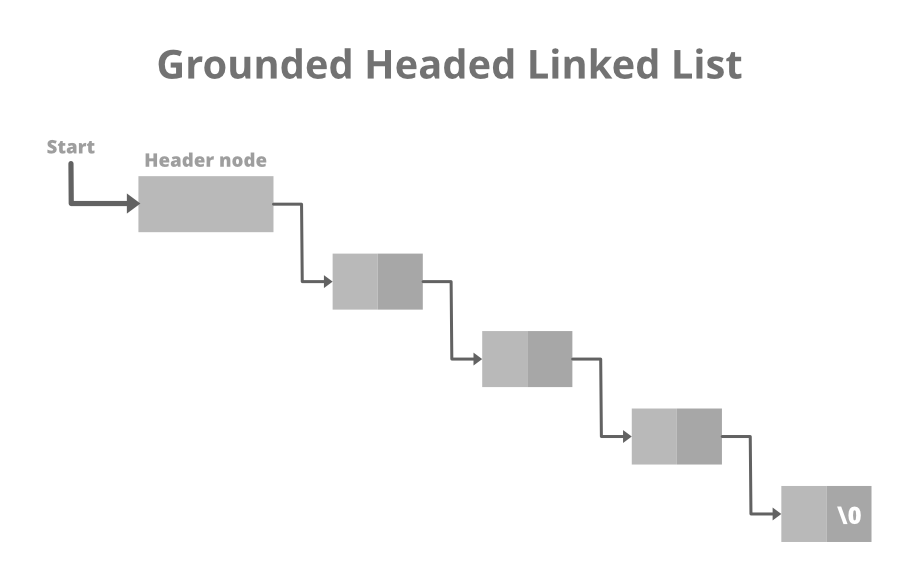## C++

 `// Structure of the list``struct` `link {``    ``int` `info;` `    ``// Pointer to the next node``    ``struct` `link* next;``};`

## Python3

 `# structure of Node``class` `Node:``    ``def` `__init__(``self``, data):``        ``self``.data ``=` `data``        ``self``.``next` `=` `None`

## Java

 `// Structure of the list``static` `class` `link {``    ``int` `info;``    ` `     ``// Pointer to the next node``    ``link next;``};` `// this code is contributed by shivanisinghss2110`

## C#

 `// Structure of the list``public` `class` `link {``    ``public` `int` `info;``    ` `    ``// Pointer to the next node``    ``public` `link next;``};` `// this code is contributed by shivanisinghss2110`

## C++

 `// C++ program to illustrate creation``// and traversal of Header Linked List``#include ``// #include ``// #include ` `// Structure of the list``struct` `link {``    ``int` `info;``    ``struct` `link* next;``};` `// Empty List``struct` `link* start = NULL;` `// Function to create header of the``// header linked list``struct` `link* create_header_list(``int` `data)``{` `    ``// Create a new node``    ``struct` `link *new_node, *node;``    ``new_node = (``struct` `link*)``        ``malloc``(``sizeof``(``struct` `link));``    ``new_node->info = data;``    ``new_node->next = NULL;` `    ``// If it is the first node``    ``if` `(start == NULL) {` `        ``// Initialize the start``        ``start = (``struct` `link*)``            ``malloc``(``sizeof``(``struct` `link));``        ``start->next = new_node;``    ``}``    ``else` `{` `        ``// Insert the node in the end``        ``node = start;``        ``while` `(node->next != NULL) {``            ``node = node->next;``        ``}``        ``node->next = new_node;``    ``}``    ``return` `start;``}` `// Function to display the``// header linked list``struct` `link* display()``{``    ``struct` `link* node;``    ``node = start;``    ``node = node->next;` `    ``// Traverse until node is``    ``// not NULL``    ``while` `(node != NULL) {` `        ``// Print the data``        ``printf``(``"%d "``, node->info);``        ``node = node->next;``    ``}``    ``printf``(``"\n"``);` `    ``// Return the start pointer``    ``return` `start;``}` `// Driver Code``int` `main()``{``    ``// Create the list``    ``create_header_list(11);``    ``create_header_list(12);``    ``create_header_list(13);` `    ``// Print the list``    ``printf``(``"List After inserting"``           ``" 3 elements:\n"``);``    ``display();``    ``create_header_list(14);``    ``create_header_list(15);` `    ``// Print the list``    ``printf``(``"List After inserting"``           ``" 2 more elements:\n"``);``    ``display();` `    ``return` `0;``}`

## Java

 `// Java program to illustrate creation``// and traversal of Header Linked List` `class` `GFG{``// Structure of the list``static` `class` `link {``    ``int` `info;``    ``link next;``};` `// Empty List``static` `link start = ``null``;` `// Function to create header of the``// header linked list``static` `link create_header_list(``int` `data)``{` `    ``// Create a new node``    ``link new_node, node;``    ``new_node = ``new` `link();``    ``new_node.info = data;``    ``new_node.next = ``null``;` `    ``// If it is the first node``    ``if` `(start == ``null``) {` `        ``// Initialize the start``        ``start = ``new` `link();``        ``start.next = new_node;``    ``}``    ``else` `{` `        ``// Insert the node in the end``        ``node = start;``        ``while` `(node.next != ``null``) {``            ``node = node.next;``        ``}``        ``node.next = new_node;``    ``}``    ``return` `start;``}` `// Function to display the``// header linked list``static` `link display()``{``    ``link node;``    ``node = start;``    ``node = node.next;` `    ``// Traverse until node is``    ``// not null``    ``while` `(node != ``null``) {` `        ``// Print the data``        ``System.out.printf(``"%d "``, node.info);``        ``node = node.next;``    ``}``    ``System.out.printf(``"\n"``);` `    ``// Return the start pointer``    ``return` `start;``}` `// Driver Code``public` `static` `void` `main(String[] args)``{``    ``// Create the list``    ``create_header_list(``11``);``    ``create_header_list(``12``);``    ``create_header_list(``13``);` `    ``// Print the list``    ``System.out.printf(``"List After inserting"``          ``+ ``" 3 elements:\n"``);``    ``display();``    ``create_header_list(``14``);``    ``create_header_list(``15``);` `    ``// Print the list``    ``System.out.printf(``"List After inserting"``          ``+ ``" 2 more elements:\n"``);``    ``display();` `}``}` `// This code is contributed by 29AjayKumar`

## Python3

 `# structure of Node``class` `Node:``    ``def` `__init__(``self``, data):``        ``self``.data ``=` `data``        ``self``.``next` `=` `None`  `class` `LinkedList:``    ``def` `__init__(``self``):``        ``self``.head ``=` `Node(``0``)``        ``self``.last_node ``=` `self``.head` `    ``# function to add elements to header linked list``    ``def` `append(``self``, data):``        ``self``.last_node.``next` `=` `Node(data)``        ``self``.last_node ``=` `self``.last_node.``next` `    ``# function to print the content of header linked list``    ``def` `display(``self``):``        ``current ``=` `self``.head.``next``        ``# traversing the header linked list``        ``while` `current ``is` `not` `None``:``            ``# at each node printing its data``            ``print``(current.data, end``=``' '``)``            ``# giving current next node``            ``current ``=` `current.``next``        ``# print(self.head.data)``        ``print``()`  `if` `__name__ ``=``=` `'__main__'``:``    ``L ``=` `LinkedList()``    ``# adding elements to the header linked list``    ``L.append(``1``)``    ``L.append(``2``)``    ``L.append(``3``)``    ``L.append(``4``)``    ``# displaying elements of header linked list``    ``L.display()`

## C#

 `// C# program to illustrate creation``// and traversal of Header Linked List` `using` `System;` `public` `class` `GFG{``// Structure of the list``public` `class` `link {``    ``public` `int` `info;``    ``public` `link next;``};` `// Empty List``static` `link start = ``null``;` `// Function to create header of the``// header linked list``static` `link create_header_list(``int` `data)``{` `    ``// Create a new node``    ``link new_node, node;``    ``new_node = ``new` `link();``    ``new_node.info = data;``    ``new_node.next = ``null``;` `    ``// If it is the first node``    ``if` `(start == ``null``) {` `        ``// Initialize the start``        ``start = ``new` `link();``        ``start.next = new_node;``    ``}``    ``else` `{` `        ``// Insert the node in the end``        ``node = start;``        ``while` `(node.next != ``null``) {``            ``node = node.next;``        ``}``        ``node.next = new_node;``    ``}``    ``return` `start;``}` `// Function to display the``// header linked list``static` `link display()``{``    ``link node;``    ``node = start;``    ``node = node.next;` `    ``// Traverse until node is``    ``// not null``    ``while` `(node != ``null``) {` `        ``// Print the data``        ``Console.Write(``"{0} "``, node.info);``        ``node = node.next;``    ``}``    ``Console.Write(``"\n"``);` `    ``// Return the start pointer``    ``return` `start;``}` `// Driver Code``public` `static` `void` `Main(String[] args)``{``    ``// Create the list``    ``create_header_list(11);``    ``create_header_list(12);``    ``create_header_list(13);` `    ``// Print the list``    ``Console.Write(``"List After inserting"``          ``+ ``" 3 elements:\n"``);``    ``display();``    ``create_header_list(14);``    ``create_header_list(15);` `    ``// Print the list``    ``Console.Write(``"List After inserting"``          ``+ ``" 2 more elements:\n"``);``    ``display();` `}``}` ` `  `// This code is contributed by 29AjayKumar`
Output
```List After inserting 3 elements:
11 12 13
List After inserting 2 more elements:
11 12 13 14 15```

My Personal Notes arrow_drop_up